# 1. 前言

## 0x1：概率论的起源

17 世纪中叶，人们开始对机会性游戏的数学规律进行探讨。它的发展与数学史上一些伟大的名字相联系，如帕斯卡、费马、惠更斯、詹姆斯、伯努利、棣莫弗、拉普拉斯等。

1654 年，费马与帕斯卡的通信中关于分赌注问题的讨论被公认为是概率论诞生的标志。问题是这样的：

“两个赌徒相约赌若干局，谁先赢 s 局就算赢了，当赌徒 A 赢 a 局 (a < s)，而赌徒 B 赢 b 局(b < s) 时，赌博被迫中止，应该怎样分配赌注才合理？”

https://baike.baidu.com/item/%E5%A4%A7%E6%95%B0%E5%AE%9A%E5%BE%8B

# 2. 伯努利大数定律（1713年）

## 0x1：数学公式定义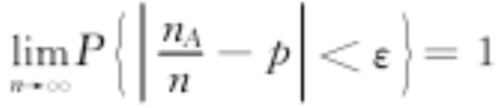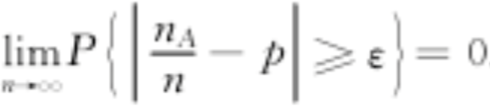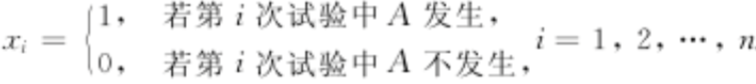A发生的频率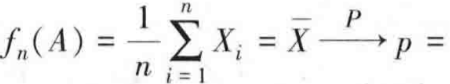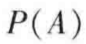https://baike.baidu.com/item/%E4%BC%AF%E5%8A%AA%E5%88%A9%E5%A4%A7%E6%95%B0%E5%AE%9A%E5%BE%8B/7922139

# 3. 棣莫弗-拉普拉斯中心极限定理（1733年）

1730 年，法国数学家棣莫弗（1677年-1754年）出版的著作《分析杂论》中包含了著名的棣莫弗─拉普拉斯定理。他使用正态分布取估计n（很大）时抛掷硬币出现正面次数的分布，即二项分布B(n，0.5)。这就是概率论中第二个基本极限定理的雏形。

## 0x1：数学公式定义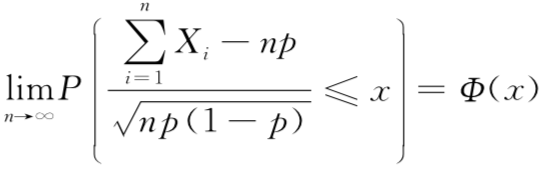• 分子就是二项分布随机变量序列的累加和，减去随机变量的期望np
• 分布就是二项分布的标准差
• 分子除分母的整个公式，可以看成是随机变量期望值标准化的过程
• 同时括号里的<=符号，表达说标准化的期望偏离0原点的概率，也即误差概率，这个偏离误差概率是符合正态分布的

## 0x2：由该定理得到二项分布的正态分布近似解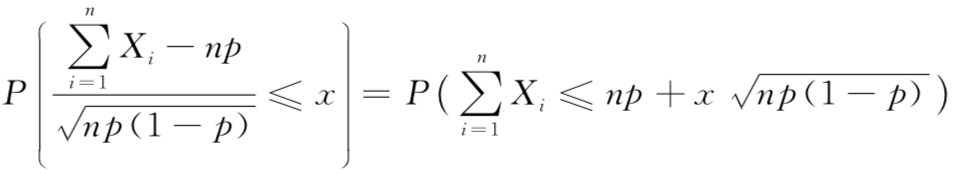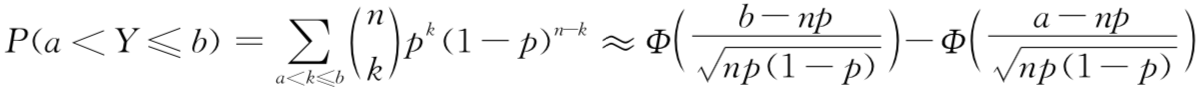## 0x3：棣莫弗-拉普拉斯中心极限定理对伯努利大数定理的更细致证明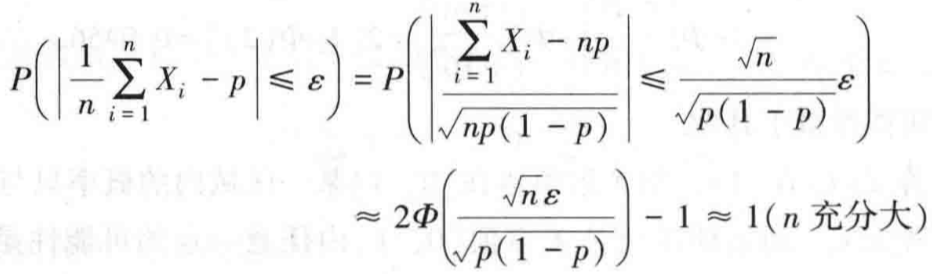## 0x4：棣莫弗-拉普拉斯定理应用举例

### 2. 解题分析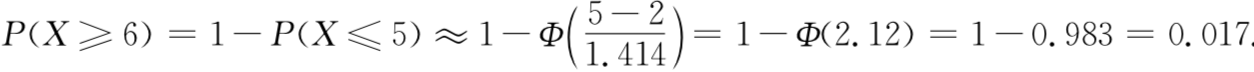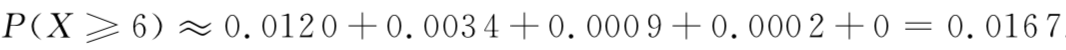https://en.wikipedia.org/wiki/De_Moivre%E2%80%93Laplace_theorem

# 4. 列维-林德伯格中心极限定理（1920年）

## 0x1：数学公式定义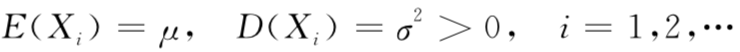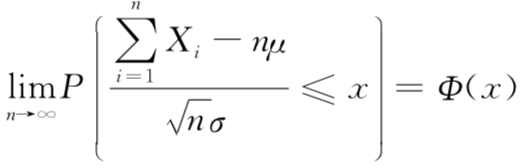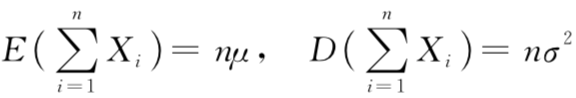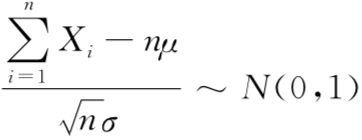或者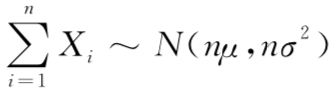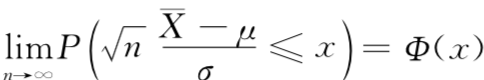## 0x2：公式的合理性分析

### 2. 高尔顿钉板实验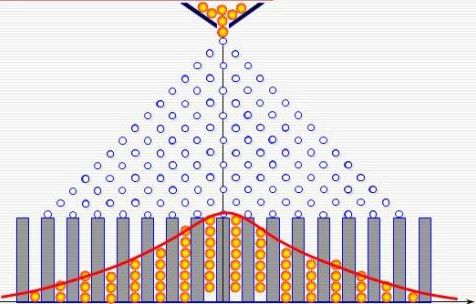• Xi = -1：小球碰到第 i 排钉子后向左下落
• Xi = 1：小球碰到第 i 排钉子后向右下落

https://baike.baidu.com/item/%E9%AB%98%E5%B0%94%E9%A1%BF%E9%92%89%E6%9D%BF/6765470
https://baike.baidu.com/item/%E6%9E%97%E5%BE%B7%E4%BC%AF%E6%A0%BC%E5%88%97%E7%BB%B4%E5%AE%9A%E7%90%86/10388983?fr=aladdin 

# 5. 辛钦大数定律（相互独立同部分大数定律）

## 0x1：数学公式定义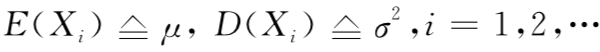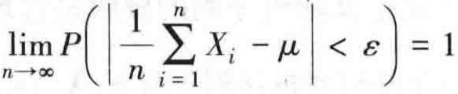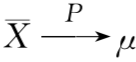## 0x2：算数平均值法则的理论依据

X1，X2，....，Xn服从同一分布，它们共同的期望记为物体的真实质量μ。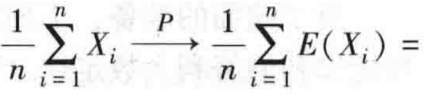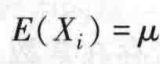https://baike.baidu.com/item/%E8%BE%9B%E9%92%A6%E5%A4%A7%E6%95%B0%E5%AE%9A%E5%BE%8B/7922188

# 6. 切比雪夫大数定律

## 0x1：切比雪夫（Chebyshev）不等式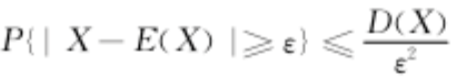• 方差越大，此上界就越大
• 方差越小，X在其期望附近取值的密集程度就越高，那么远离期望的区域的概率上界就越小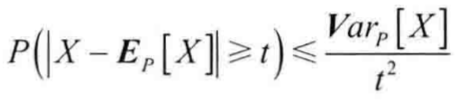，令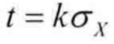，则有，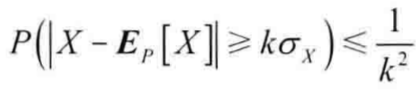## 0x2：依概率收敛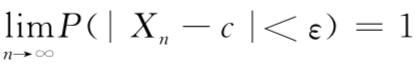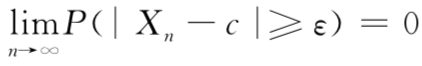## 0x3：切比雪夫大数定律数学公式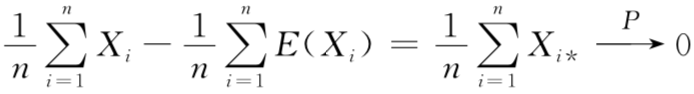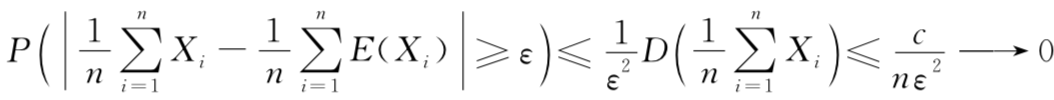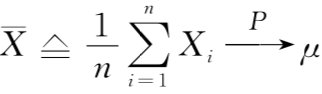## 0x4：三个大数定律之间的条件关系

• 切比雪夫大数定律不要求随机变量序列同分布，甚至不要求相互独立，只要两两不线性相关、方差一致有界即可
• 辛钦大数定律要求随机变量相互独立且同分布，但不要求方差存在，仅期望存在即可
• 努利大数定律要求随机变量相互独立且同分布，但限定于伯努利两点分布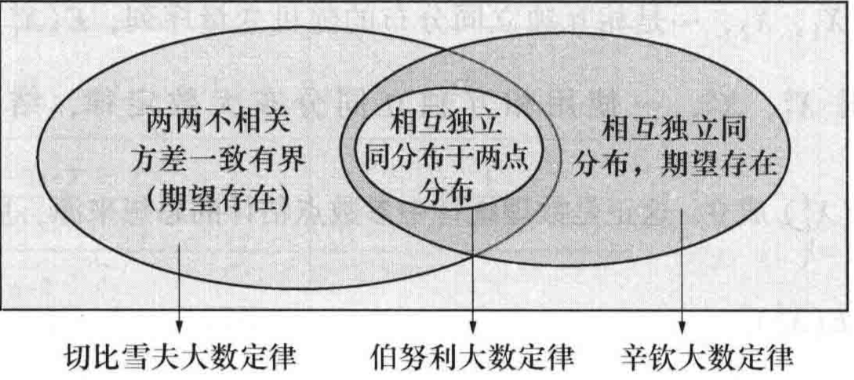# 7.  大数定律和中心极限定理的异同

## 0x1：中心极限定理和大数定律的区别

• 大数定律是说，n只要越来越大，把这n个独立同分布的数加起来去除以n得到的这个样本均值（也是一个随机变量）会依概率收敛到真值u，但是样本均值的分布是怎样的我们不知道
• 中心极限定理是说，n只要越来越大，这n个数的样本均值会趋近于正态分布，并且这个正态分布以u为均值，sigma^2/n为方差
• 综上所述，这两个定律都是在说样本均值性质。随着n增大，大数定律说样本均值几乎必然等于均值。中心极限定律说，它越来越趋近于正态分布，并且这个正态分布的方差越来越小

## 0x2：中心极限定理在概率论中的核心地位# 8. 大数定律的理论应用

• 1）算术平均法则
• 2）频率估计概率方法
• 3）数理统计中参数的点估计思想，这也是很多传统机器学习模型训练的理论支撑
• 4）中心极限定理在数理统计的区间估计与假设检验问题中的应用

# 9. 大数定律存在的一个实验例证

## 0x1：泊松随机变量的收敛 - 在大数N时，大数定理普遍存在的一个例证

# -*- coding: utf-8 -*-

import numpy as np
from IPython.core.pylabtools import figsize
import matplotlib.pyplot as plt

figsize(12.5, 5)
import pymc as pm

sample_size = 100000
expected_value = lambda_ = 4.5
poi = pm.rpoisson
N_samples = range(1, sample_size, 100)
print N_samples

for k in range(3):

samples = poi(lambda_, size=sample_size)

partial_average = [samples[:i].mean() for i in N_samples]   # 从[1:sample_size]不同样本数下，三个泊松分布的均值

plt.plot(N_samples, partial_average, lw=1.5, label="average \
of  $n$ samples; seq. %d" % k)

plt.plot(N_samples, expected_value * np.ones_like(partial_average),
ls="--", label="true expected value", c="k")

plt.ylim(4.35, 4.65)
plt.title("Convergence of the average of \n random variables to its \
expected value")
plt.ylabel("average of $n$ samples")
plt.xlabel("# of samples, $n$")
plt.legend()

plt.show()### 1. 收敛到期望值的速度

#### 1）通过实验方式来观察收敛速度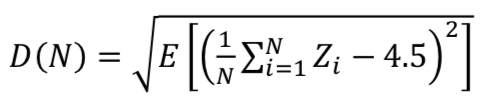# -*- coding: utf-8 -*-

import numpy as np
from IPython.core.pylabtools import figsize
import matplotlib.pyplot as plt
import pymc as pm

figsize(12.5, 4)

sample_size = 100000
expected_value = lambda_ = 4.5
poi = pm.rpoisson
N_samples = range(1, sample_size, 100)

N_Y = 250  # use this many to approximate D(N)
N_array = np.arange(1000, 50000, 2500)  # use this many samples in the approx. to the variance.
D_N_results = np.zeros(len(N_array))

def D_N(n):
"""
This function approx. D_n, the average variance of using n samples.
"""
Z = poi(lambda_, size=(n, N_Y))
average_Z = Z.mean(axis=0)
return np.sqrt(((average_Z - expected_value) ** 2).mean())

for i, n in enumerate(N_array):
D_N_results[i] = D_N(n)

plt.xlabel("$N$")
plt.ylabel("expected squared-distance from true value")
plt.plot(N_array, D_N_results, lw=3,
label="expected distance between\n\
expected value and \naverage of $N$ random variables.")
plt.plot(N_array, np.sqrt(expected_value) / np.sqrt(N_array), lw=2, ls="--",
label=r"$\frac{\sqrt{\lambda}}{\sqrt{N}}$")
plt.legend()
plt.title("How 'fast' is the sample average converging? ")

plt.show()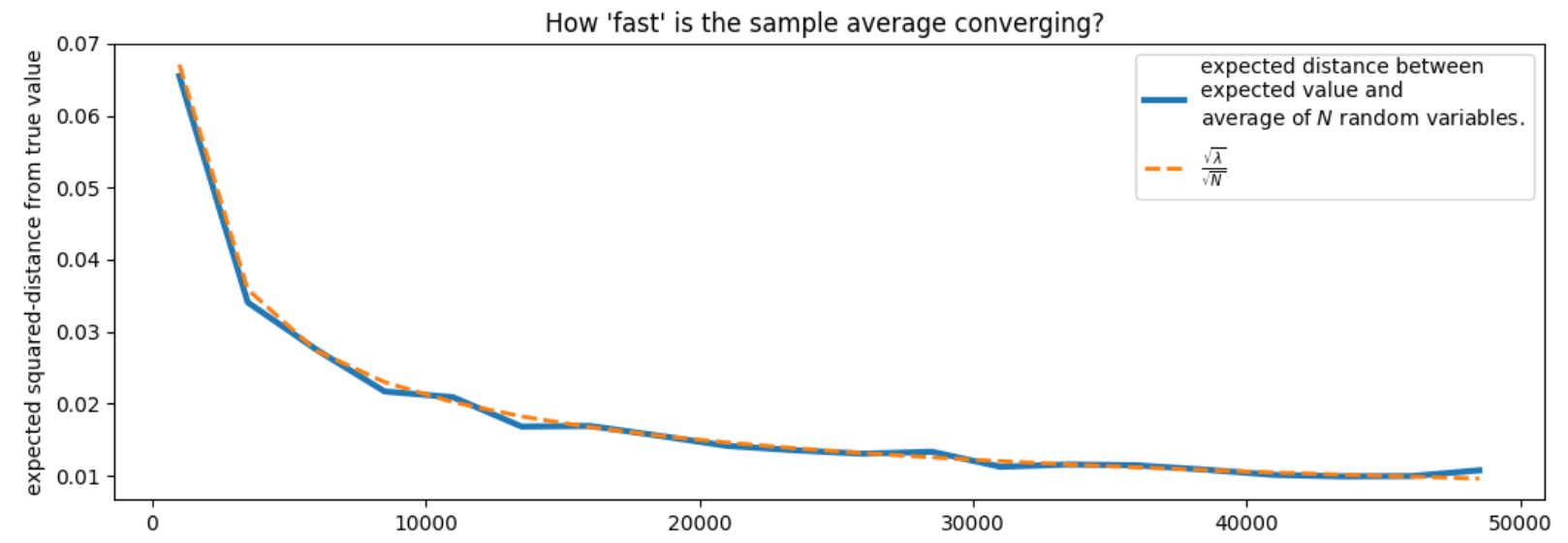### 2. 连接概率和频率之间的桥梁 - 大数定理

频率统计 <---->(大数定理) <----> 期望 <----> 概率

## 0x2：小数据的无序性 - 当 N 很小时大数定理会失效

### 1. 实例1 - 地理数据的聚合

# -*- coding: utf-8 -*-

import numpy as np
from IPython.core.pylabtools import figsize
import matplotlib.pyplot as plt
import pymc as pm

figsize(12.5, 4)
std_height = 15
mean_height = 150

n_counties = 5000
pop_generator = pm.rdiscrete_uniform
norm = pm.rnormal

# generate some artificial population numbers
population = pop_generator(100, 1500, size=n_counties)

average_across_county = np.zeros(n_counties)
for i in range(n_counties): # 5000个县
# generate some individuals and take the mean
average_across_county[i] = norm(mean_height, 1. / std_height ** 2,  # 每个县都服从相同的分布: height~Normal(150,15)
size=population[i]).mean()　　# 不同的县人口

# located the counties with the apparently most extreme average heights.
i_min = np.argmin(average_across_county)
i_max = np.argmax(average_across_county)

# plot population size vs. recorded average
plt.scatter(population, average_across_county, alpha=0.5, c="#7A68A6")
plt.scatter([population[i_min], population[i_max]],
[average_across_county[i_min], average_across_county[i_max]],
s=60, marker="o", facecolors="none",
edgecolors="#A60628", linewidths=1.5,
label="extreme heights")

plt.xlim(100, 1500)
plt.title("Average height vs. County Population")
plt.xlabel("County Population")
plt.ylabel("Average height in county")
plt.plot([100, 1500], [150, 150], color="k", label="true expected \
height", ls="--")
plt.legend(scatterpoints=1)

plt.show()### 2. 实例2 - Kaggle的美国人口普查反馈比例预测

# -*- coding: utf-8 -*-

import numpy as np
from IPython.core.pylabtools import figsize
import matplotlib.pyplot as plt
import pymc as pm

figsize(12.5, 6.5)
delimiter=",")
plt.scatter(data[:, 1], data[:, 0], alpha=0.5, c="#7A68A6")
plt.title("Census mail-back rate vs Population")
plt.ylabel("Mail-back rate")
plt.xlabel("population of block-group")
plt.xlim(-100, 15e3)
plt.ylim(-5, 105)

i_min = np.argmin(data[:, 0])
i_max = np.argmax(data[:, 0])

plt.scatter([data[i_min, 1], data[i_max, 1]],
[data[i_min, 0], data[i_max, 0]],
s=60, marker="o", facecolors="none",
edgecolors="#A60628", linewidths=1.5,
label="most extreme points")

plt.legend(scatterpoints=1)

plt.show()### 3. 实例3 - 如何对Reddit网站上的评论进行排序

#### 1）我们如何对评论进行排序

Reddit是一个流行的网站。它主要提供一些小说或者图片的链接，这个网站最流行的部分是它对每个链接的评论。Reddit可以对每个评论进行投票(被称为赞成票或否决票)。默认状态下Reddit将把最好的评论排在前面。

#### 2）真实的好评率如何得到？ - 贝叶斯推断来帮忙

1. 倾斜数据: 大部分的评论都没有投票数据，因此有许多评论的好评率都非常小 (极端值)，这就使分布大多数集中在极端值附件。可以为投票的个数设个阈值，大于 该阈值的情况才予以考虑。阈值的选择还要考虑其对好评率精度的影响。
2. 偏差数据: Reddit有许多不同的子页面，称为subreddits。例如有两个页面，第一个为:r/aww 页面粘贴许多可爱动物的照片，第二个为:r/politics 讨论政治问题。用户对这两个页面的评论行为是不 相同的。浏览第一个页面的人的性格会更友好，更有爱心，也就会有更多的好评(与第二个页面相比)，而第二个页面的评论可能会有更多的争论或不同的观点出现。因此并不是所有的评论都是一致的。

import pymc as pm

"""
and the number of posterior samples to return to the user. Assumes a uniform prior.
"""
upvote_ratio = pm.Uniform("upvote_ratio", 0, 1)
observations = pm.Binomial("obs", N, upvote_ratio, value=upvotes, observed=True)
# do the fitting; first do a MAP as it is cheap and useful.
map_ = pm.MAP([upvote_ratio, observations]).fit()
mcmc = pm.MCMC([upvote_ratio, observations])
mcmc.sample(samples, samples / 4)
return mcmc.trace("upvote_ratio")[:]

figsize(11., 8)
posteriors = []
colours = ["#348ABD", "#A60628", "#7A68A6", "#467821", "#CF4457"]
for i in range(len(submissions)):
j = submissions[i]
plt.hist(posteriors[i], bins=18, normed=True, alpha=.9,
histtype="step", color=colours[i % 5], lw=3,
plt.hist(posteriors[i], bins=18, normed=True, alpha=.2,
histtype="stepfilled", color=colours[i], lw=3, )

plt.legend(loc="upper left")
plt.xlim(0, 1)
plt.title("Posterior distributions of upvote ratios on different submissions")#### 3）如何针对评论的后验分布的进行排序

N = posteriors.shape
lower_limits = []

for i in range(len(submissions)):
j = submissions[i]
plt.hist(posteriors[i], bins=20, normed=True, alpha=.9,
histtype="step", color=colours[i], lw=3,
plt.hist(posteriors[i], bins=20, normed=True, alpha=.2,
histtype="stepfilled", color=colours[i], lw=3, )
v = np.sort(posteriors[i])[int(0.05 * N)]
# plt.vlines( v, 0, 15 , color = "k", alpha = 1, linewidths=3 )
plt.vlines(v, 0, 10, color=colours[i], linestyles="--", linewidths=3)
lower_limits.append(v)
plt.legend(loc="upper left")

plt.legend(loc="upper left")
plt.title("Posterior distributions of upvote ratios on different submissions");
order = np.argsort(-np.array(lower_limits))
print(order, lower_limits)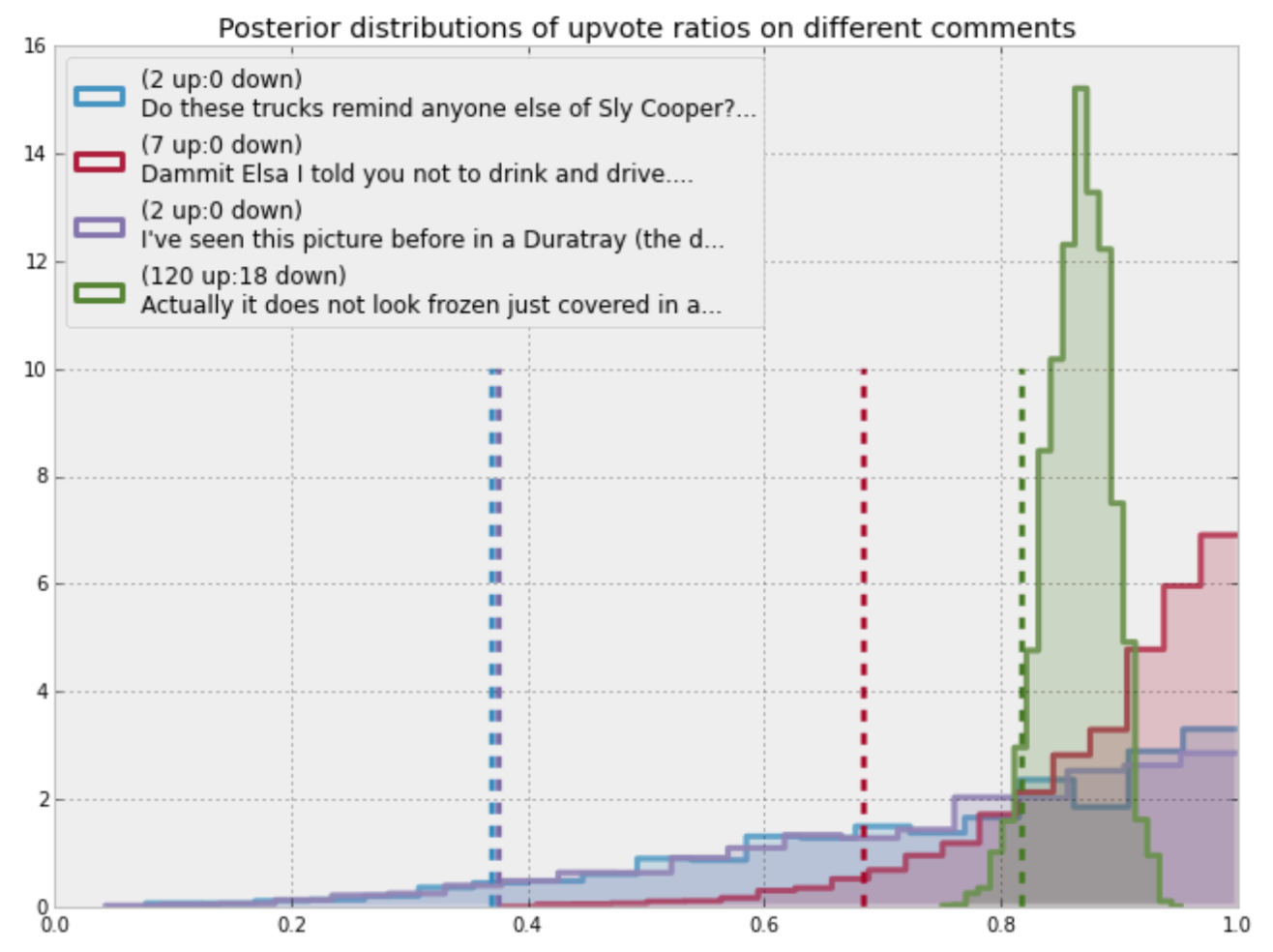1. 给定两个好评率相同的评论，我们会选择票数最多的作为更加评论，因为票数更多的评论在贝叶斯后验概率分布中更集中，落在 95%置信区间的概率也更大，也即我们更确定其好评率更好。
2. 给定两个票数一样的评论，我们会选择好评数更多的。

# 10. 大数定律在工程领域的具体应用 - 保险行业的赚钱原理

## 0x2：保费制定的理论支撑 - 大数定律

nb = a * E(X) = a * np

=> b = ap

# 11. 我们怎样更好应用大数定理

1. 通过（低成本地）获得大量后验样本，可以确保大数定理适用于期望的近似估计
2. 在样本数量很小的贝叶斯推断中，后验分布包括更多的随机性。从后验分布中将能看出这些随机性的存在。后验分布越平坦随机性越大，越陡峭随机性越小。因此推断的结果是需要调校的。
3. 不考虑样本量会带来很大的影响，此时排序的依据往往是不稳定的，并会导致非常病态的排序结果。

posted @ 2018-09-02 11:27  郑瀚Andrew.Hann  阅读(2908)  评论(0编辑  收藏  举报